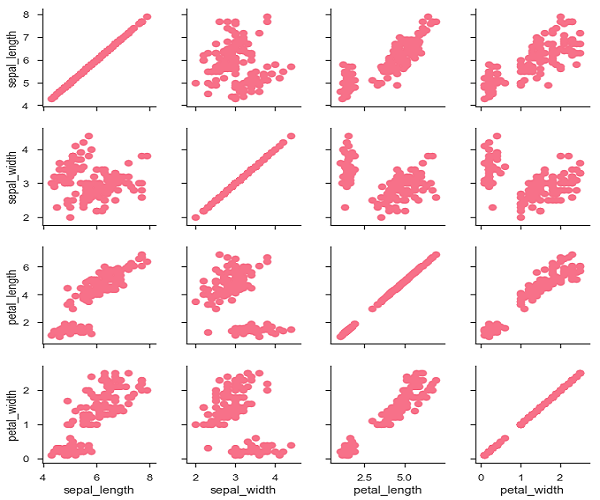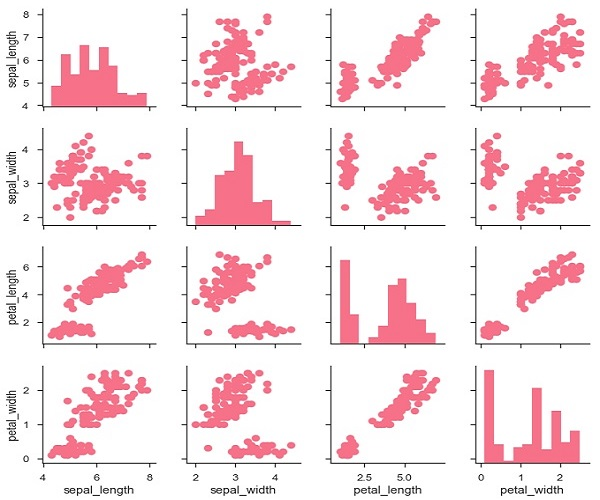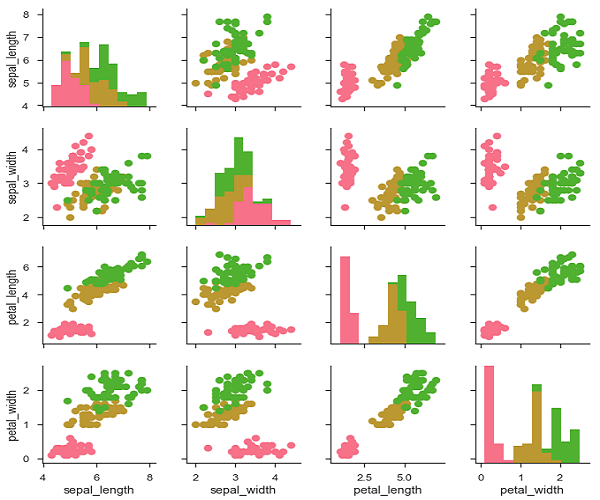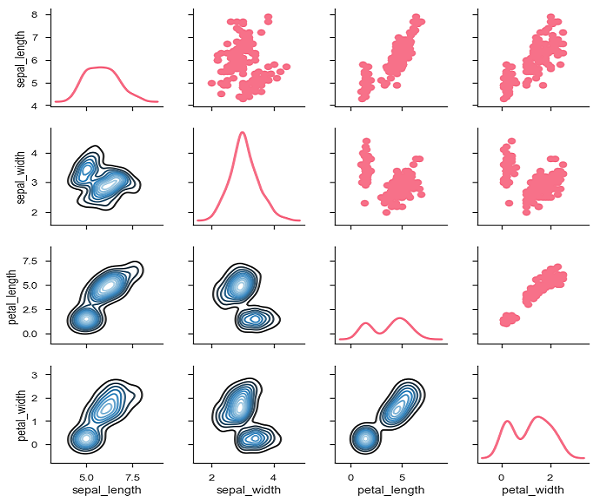Seaborn - Pair Grid

PairGrid allows us to draw a grid of subplots using the same plot type to visualize data.

Unlike FacetGrid, it uses different pair of variable for each subplot. It forms a matrix of sub-plots. It is also sometimes called as “scatterplot matrix”.

The usage of pairgrid is similar to facetgrid. First initialise the grid and then pass the plotting function.

Example

import pandas as pd
import seaborn as sb
from matplotlib import pyplot as plt
g = sb.PairGrid(df)
g.map(plt.scatter);
plt.show()It is also possible to plot a different function on the diagonal to show the univariate distribution of the variable in each column.

Example

import pandas as pd
import seaborn as sb
from matplotlib import pyplot as plt
g = sb.PairGrid(df)
g.map_diag(plt.hist)
g.map_offdiag(plt.scatter);
plt.show()

OutputWe can customize the color of these plots using another categorical variable. For example, the iris dataset has four measurements for each of three different species of iris flowers so you can see how they differ.

Example

import pandas as pd
import seaborn as sb
from matplotlib import pyplot as plt
g = sb.PairGrid(df)
g.map_diag(plt.hist)
g.map_offdiag(plt.scatter);
plt.show()

OutputWe can use a different function in the upper and lower triangles to see different aspects of the relationship.

Example

import pandas as pd
import seaborn as sb
from matplotlib import pyplot as plt
g = sb.PairGrid(df)
g.map_upper(plt.scatter)
g.map_lower(sb.kdeplot, cmap = "Blues_d")
g.map_diag(sb.kdeplot, lw = 3, legend = False);
plt.show()

OutputUseful Video Courses

Video

Data Visualization using MatPlotLib & Seaborn

11 Lectures 4 hours

Video

Seaborn with Python

11 Lectures 2.5 hours